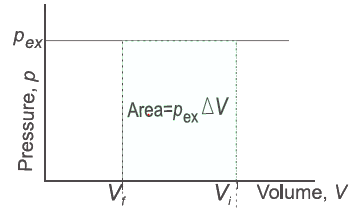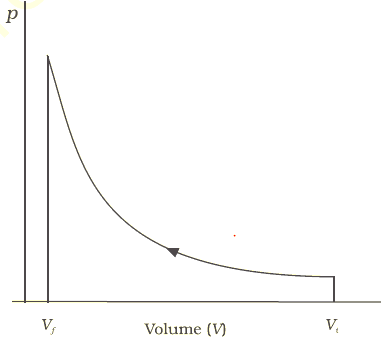# Thermodynamics And Types of processes

## Thermodynamics

Science which deals with study of different forms of energy and quantitative relationship

## System & Surrounding

The part of universe for study is called system and remaining portion is surroundings.
System and Surrounding together constitute Universe
System + surrounding = Universe

## Types of System

### Open System

In a system when there is exchange of matter and energy taking place with the surrounding.

### Closed System

In a system when there is exchange of energy only and not the matter with the surrounding.

### Isolated System

In a system when there is no exchange of energy and matter taking place with the surrounding.

## Homogenous System

When all the constituents present in the systems are in the same face and is uniform throughout the systems.
Example Mixture of two miscible liquids.

## Hetrogenous System

A mixture is said to be nitrogenous when it consists of two or more phases and its composition is not uniform throughout the system.
Example Sand and Water

## State of a System & State Function

It means its macroscopic or bulk properties which can be described by state variation – pressure, Volume, temperature, etc. They are also known as state function.

## TYPES OF PROCESSES

Isothermal
The process carried out at constant temperature is known as Isothermal process
ΔT = 0
Isochoric
Process carried out at constant volume
ΔV = 0
Isobaric
Process carried out at constant process
ΔP = 0
Process carried out at constant heat (there is no transfer of heat between system & surrounding)
Δq = 0
q -> Heat
Cyclic Process
When a system undergoes a series of changes and finally return to its initial stage and is known as cyclic process.
Reversible Process
When in a process a change is brought in such a way that process would at any moment be reversed by a small change.A reversible process proceeds infinitely slowly by a series of equilibrium states such that system and the surroundings are always in near equilibrium with each other
Irreversible Process
Processes which does not follow the the reversible process are called irreversible process

## Extensive & Intensive Properties

Properties which depends on quantity of matter called extensive prop. Example, mass, volume, heat capacity, enthalpy, entropy etc. The properties which do not depend on quantity of matter present are called Intensive properties. Example, T, P, density, refractive index, viscosity, Boiling point, pH, mole fraction etc.

## Internal Energy (u)

It is sum of all form of energies that a system can process.
i.e. U = Ee + En + Ec + Ep + Ek + ------
ΔU = U2 – U1 or Uf – Ui & U is state function and extensive properly.
If U1> U2 energy is released and if U1 < U2, energy is absorbed

## IUPAC rules for Chemical thermodynamics

Change in Internal Energy by work
Work done by the system(W) = negative
Work done on the system(W) = positive
Change in Internal Energy by doing work
ΔU = UB – UA = W

Change in Internal Energy by Transfer of Heat
Internal Energy is changed by transfer of heat from surrounding to the system without doing work.
ΔU = q
When the heat is absorbed by the system it is denoted by ‘q’ and measured in terms of temperature difference.
When heat is transferred from the surrounding to the system
q = +ve
When heat is transferred from system to surrounding
q = -ve
If change of state is done by doing both work & transfer of heat then
ΔU = q + W
Where
W -> work done
q -> Heat Transfer

## Zeroth (0th) Law of Thermodynamics

According to this law if two systems are in thermal equilibrium with 3rd system. They are also in thermal equilibrium with each other.

## First law of thermodynamics

According to this Energy can neither be created nor be destroyed but energy can change from one form to another.
Mathematical form:
If U1 = Initial Internal Energy
Q= heat change
W= work done
Then U2 = Final Internal energy is given by
U2 = U1 + q + W
U2 – U1 = q + W
ΔU = q + w

## Workdone

If pressure of gas is P and external pressure is pext. then
Force = Pressure  × Area
So, Work done = Force × L
= P× A ×L
= -Pext ΔV
Negative sign is placed to get the appropriate sign for work. So, in case of compression i.e.  work done on the  system will be positive and in case of expansion ,work done by the system will be negative
This can be represented in the PV graph as belowFor reversible process, it can be written as
$W=-\int_{V_i}^{V_f}P_{ext}\Delta V$
Now Pext = P ±dP
$W=-\int_{V_i}^{V_f}(P+dP) \Delta V$
$W=-\int_{V_i}^{V_f}P \Delta V$
Where P =nRT/V
This can be represented in the PV graph as belowWork done in isothermal and reversible expansion of gas
W = - nRT ln(V2 /V1)
W = -2.303 nRT log(V2 /V1)
Now  ΔU=0  for Isothermal Process
So ,
Q =-W = 2.303 nRT log(V2 /V1)
Isothermal and free expansion of Ideal gas for Isothermal expansion of an ideal gas into Vacuum
Work done = 0 as Pext =0
Since, External pressure = 0
u = 0 and q = 0
For isothermal irreversible change
W=-Pext ΔV
Q=Pext ΔV

## Solved Examples

Question 1
Five litres of an ideal gas at a pressure of  10 atm expands isothermally at 100 C into a vacuum until its total volume is 10 litres.
a. How much heat is absorbed and how much work is done in the expansion ?
b. How much heat is absorbed and how much work is done in the expansion when constant external pressure
of 1 atm exists
Solution
a. Here Pext =0
W=0 and q=0
b. Here Pext =1
q = – w = Pext (Vf -Vi) = 5 litre-atm
Question 2
A sample of gas expands isothermally from 10L to 20L against pressure of 2 atm.Calculate the work done.
Solution
W=-Pext ΔV
=2 (20 – 10)
= -20 litre-atm
Question 3
A balloon contains 10 moles of a gas in a vol. Of 5l at 27oc. Balloon burst so that gas expands against 1 atm. Pressure. Calculate the work done by the gas. (R = 0.0821)
Solution
n = 10
PV = nRT
1  × V = 10 ×.0821 ×300
V = 8.21 ×.300=246.31L
Vin = 5L
Work done =-Pext ΔV
= -1(246.31-5)
= - 241.3 joules